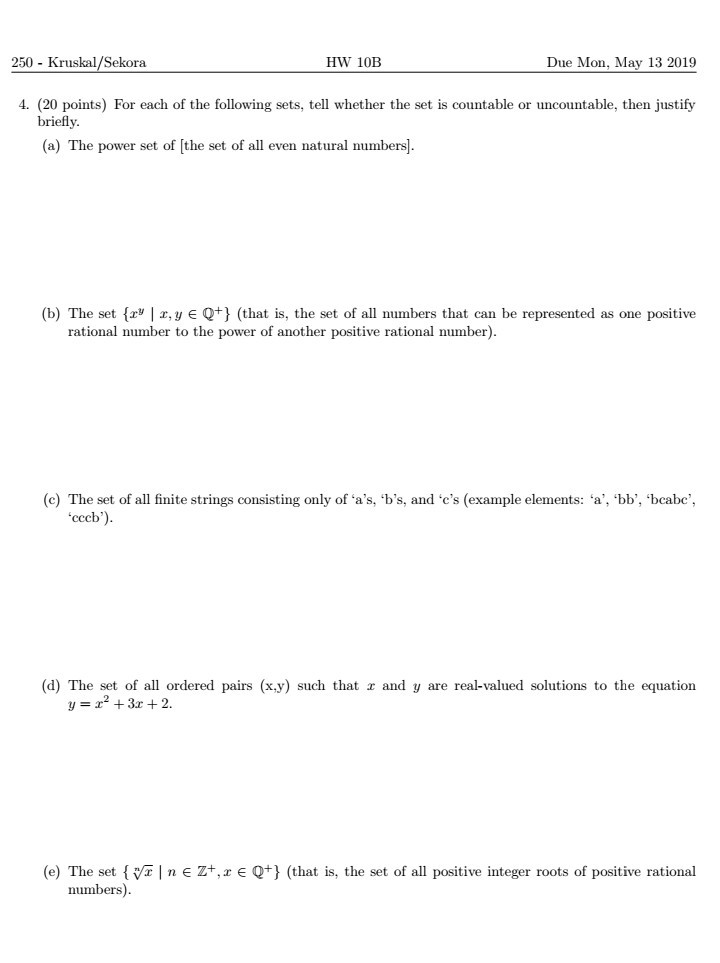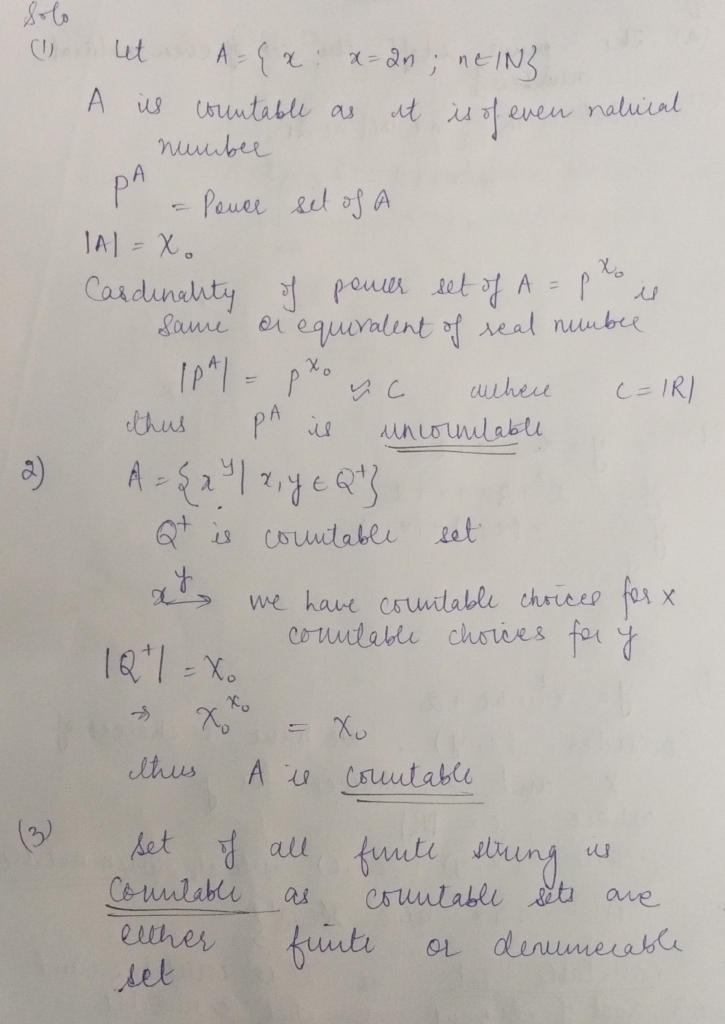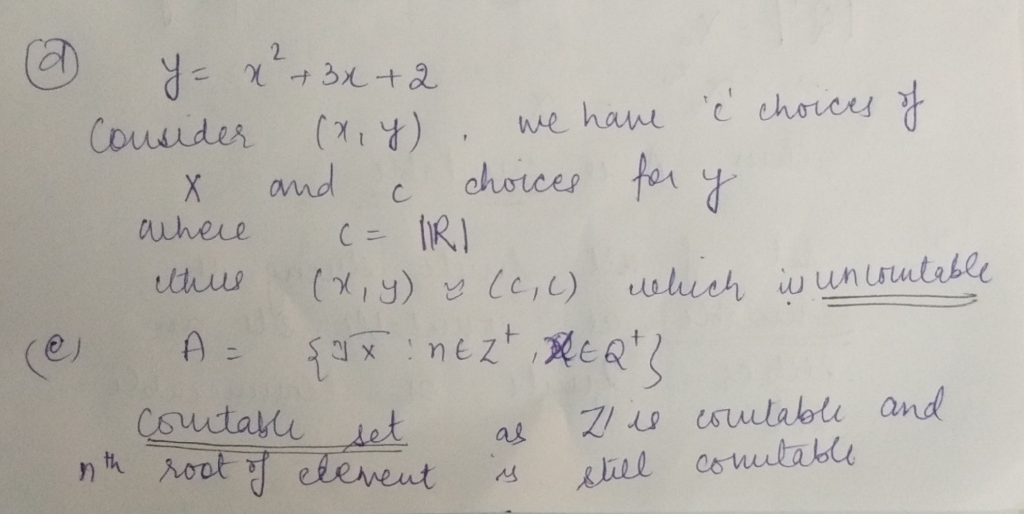Homework Help Question & Answers

250- Kruskal/Sekora HW 10B Due Mon, May 13 201 4. (20 points) For each of the following sets, tell whether the set is countable or uncountable, then justify briefly (a) The power set of [the set o...250- Kruskal/Sekora HW 10B Due Mon, May 13 201 4. (20 points) For each of the following sets, tell whether the set is countable or uncountable, then justify briefly (a) The power set of [the set of all even natural numbers) (b) The set fr x, y EQ+ (that is, the set of all numbers that can be represented as one positive rational number to the power of another positive rational number) consisting only of a's, (c) The set of all finite strings c 'b's, and 'c's (example elements: 'a', 'bb bcabc', cccb (d) The set of all ordered pairs (x.y) such that z and y are real-valued solutions to the equation y=x2 + 3x + 2. (e) The set lvrIneZ+, eQ+ (that is, the set of all positive integer roots of positive rational numbers)Add Answer of: 250- Kruskal/Sekora HW 10B Due Mon, May 13 201 4. (20 points) For each of the following sets, tell whether the set is countable or uncountable, then justify briefly (a) The power set of [the set o...
More Homework Help Questions Additional questions in this topic.

• 6.2.24 Justify each Assume all vectors are in R. Mark each statement True or False. Justify each answer a. Not every orthogonal set in Rn is linearly independent. O A. False. Orthogonal sets must be...

Need Online Homework Help?

Get FREE EXPERT Answers
WITHIN MINUTES
Related Questions